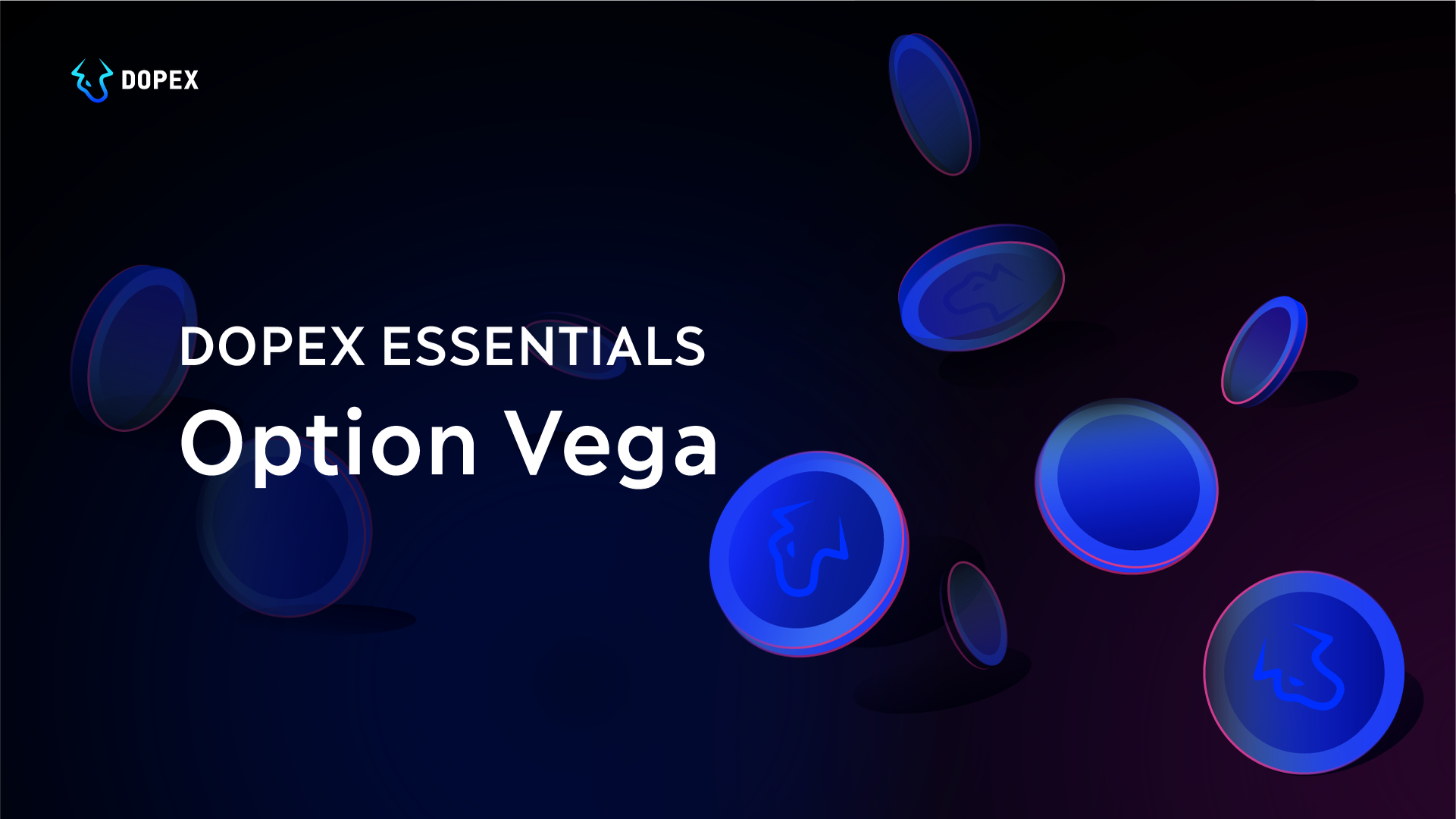# Dopex Essentials: Option Vega

Dopex-Essentials###### As an options trader you are exposed to the following risks:
• Delta Risk (Directional risk): Changes in the underlying asset price
• Gamma Risk: Changes in the directional risk of a position
• Theta Risk (Theta/Time Decay): The passage of time
• Vega Risk (Volatility): Changes in implied volatility

# Vega

## Key Points

• Due to changes in implied volatility, the value of vega can fluctuate even without price changes to the underlying asset.
• Vega can increase in reaction to sudden changes in the price of the underlying asset.
• As the option gets closer to the expiration date, the value of vega decreases.

# Conclusion

• Vega for all options is always a positive number because options increase in value when volatility increases and decrease in value when volatility decreases.
• When position Vegas are generated, however, positive and negative signs appear. When you establish a position selling or buying an option, this will result in either a negative sign (for selling) or positive sign (for buying), and the position Vega will depend on the sum of all the individual Vegas.
• Vega is always the same value for puts as for calls.
• Option prices always increase as the volatility does.
• As vega becomes smaller, volatility has less effect on the option price.
• The size of vega itself mainly depends on the relative value between the underlying asset price and the strike price and on the time to expiry of the option.
• Due to changes in implied volatility, the value of vega can fluctuate even without price changes to the underlying asset.
• Vega can increase in reaction to sudden changes in the price of the underlying asset.
• As the option gets closer to the expiration date, the value of vega decreases.
• Vega is higher on options that have more distant expiration dates. However, since those options have higher premiums, the vega is actually higher on options with closer expiration if we look at percentage gain or loss.

# Glossary of terms

###### In the money (ITM):
• For a call — this term is used when the strike price is lower than the current price of the underlying asset.
• For a put — this term is used when the strike is higher than the current price.
###### At the money (ATM):
• For both a call and a put — this term is used when the strike is equal to the current price.
###### Out of the money (OTM):
• For a call — this term is used when the strike price is higher than the current price of the underlying asset.
• For a put — this term is used when the strike is lower than the current price.

← Back to Blog